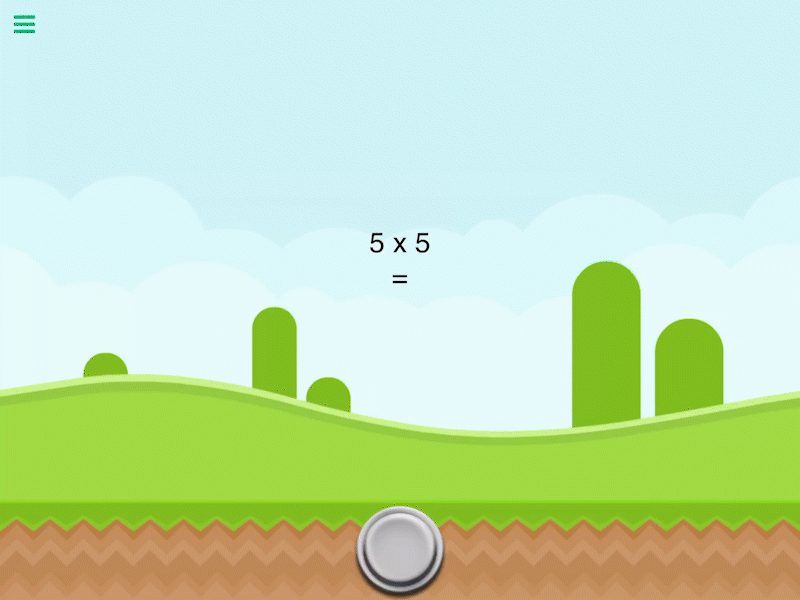# Multiply Values

Description

Multiply a number by another number. This will output the product of the 2 numbers entered.

Outputs

 Multiply Values Outputs the product of two inputs.

Examples

2 multiplied by 2 outputs 4.

Related

Divide Values

Modulus

Square Root

Subtract Values